Download Presentation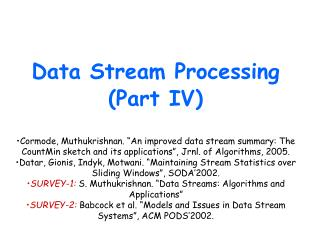Data Stream Processing (Part IV)

# Data Stream Processing (Part IV) - PowerPoint PPT Presentation

Data Stream Processing (Part IV). Cormode, Muthukrishnan. “An improved data stream summary: The CountMin sketch and its applications”, Jrnl. of Algorithms, 2005. Datar, Gionis, Indyk, Motwani. “Maintaining Stream Statistics over Sliding Windows”, SODA’2002.I am the owner, or an agent authorized to act on behalf of the owner, of the copyrighted work described.
Download Presentation## Data Stream Processing (Part IV)

An Image/Link below is provided (as is) to download presentation

Download Policy: Content on the Website is provided to you AS IS for your information and personal use and may not be sold / licensed / shared on other websites without getting consent from its author.While downloading, if for some reason you are not able to download a presentation, the publisher may have deleted the file from their server.

- - - - - - - - - - - - - - - - - - - - - - - - - - E N D - - - - - - - - - - - - - - - - - - - - - - - - - -
Presentation Transcript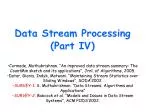### Data Stream Processing(Part IV)

• Cormode, Muthukrishnan. “An improved data stream summary: The CountMin sketch and its applications”, Jrnl. of Algorithms, 2005.
• Datar, Gionis, Indyk, Motwani. “Maintaining Stream Statistics over Sliding Windows”, SODA’2002.
• SURVEY-1: S. Muthukrishnan. “Data Streams: Algorithms and Applications”
• SURVEY-2: Babcock et al. “Models and Issues in Data Stream Systems”, ACM PODS’2002.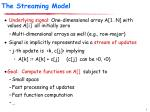The Streaming Model
• Underlying signal: One-dimensional array A[1…N] with values A[i] all initially zero
• Multi-dimensional arrays as well (e.g., row-major)
• Signal is implicitly represented via a stream of updates
• j-th update is <k, c[j]> implying
• A[k] := A[k] + c[j] (c[j] can be >0, <0)
• Goal: Compute functions on A[] subject to
• Small space
• Fast processing of updates
• Fast function computation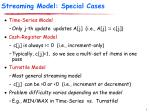Streaming Model: Special Cases
• Time-Series Model
• Only j-th update updates A[j] (i.e., A[j] := c[j])
• Cash-Register Model
• c[j] is always >= 0 (i.e., increment-only)
• Typically, c[j]=1, so we see a multi-set of items in one pass
• Turnstile Model
• Most general streaming model
• c[j] can be >0 or <0 (i.e., increment or decrement)
• Problem difficulty varies depending on the model
• E.g., MIN/MAX in Time-Series vs. Turnstile!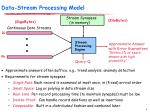Data-StreamProcessingModel

Stream Synopses

(in memory)

(KiloBytes)

• Approximate answers often suffice, e.g., trend analysis, anomaly detection
• Requirements for stream synopses
• Single Pass: Each record is examined at most once, in (fixed) arrival order
• SmallSpace: Log or polylog in data stream size
• Real-time: Per-record processing time (to maintain synopses) must be low
• Delete-Proof: Can handle record deletions as well as insertions
• Composable: Built in a distributed fashion and combined later

(GigaBytes)

Continuous Data Streams

R1

Stream

Processing

Engine

with Error Guarantees

“Within 2% of exact

probability”

Rk

Query Q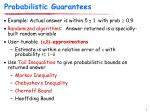Probabilistic Guarantees
• Example: Actual answer is within 5 ± 1 with prob  0.9
• Randomized algorithms: Answer returned is a specially-built random variable
• User-tunable (e,d)-approximations
• Estimate is within a relative error of e with probability >= 1-d
• Use Tail Inequalities to give probabilistic bounds on returned answer
• Markov Inequality
• Chebyshev’s Inequality
• Chernoff Bound
• Hoeffding Bound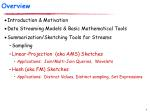Overview
• Introduction & Motivation
• Data Streaming Models & Basic Mathematical Tools
• Summarization/Sketching Tools for Streams
• Sampling
• Linear-Projection (aka AMS) Sketches
• Applications: Join/Multi-Join Queries, Wavelets
• Hash (aka FM) Sketches
• Applications: Distinct Values, Distinct sampling, Set Expressions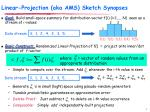2

2

1

1

1

f(1) f(2) f(3) f(4) f(5)

Data stream: 3, 1, 2, 4, 2, 3, 5, . . .

Data stream: 3, 1, 2, 4, 2, 3, 5, . . .

Linear-Projection (aka AMS) Sketch Synopses
• Goal: Build small-space summary for distribution vector f(i) (i=1,..., N) seen as a stream of i-values
• Basic Construct:Randomized Linear Projection of f() = project onto inner/dot product of f-vector
• Simple to compute over the stream: Add whenever the i-th value is seen
• Generate ‘s in small (logN) space using pseudo-random generators
• Tunable probabilistic guarantees on approximation error
• Delete-Proof: Just subtract to delete an i-th value occurrence
• Composable: Simply add independently-built projections

where = vector of random values from an appropriate distribution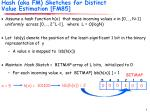BITMAP

5 4 3 2 1 0

lsb(h(x)) = 2

h(x) = 101100

0

0

0

1

0

0

Hash (aka FM) Sketches for Distinct Value Estimation [FM85]
• Assume a hash function h(x) that maps incoming values x in [0,…, N-1] uniformly across [0,…, 2^L-1], where L = O(logN)
• Let lsb(y) denote the position of the least-significant 1 bit in the binary representation of y
• A value x is mapped to lsb(h(x))
• Maintain Hash Sketch = BITMAP array of L bits, initialized to 0
• For each incoming value x, set BITMAP[ lsb(h(x)) ] = 1

x = 5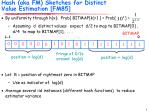BITMAP

0

0

0

1

0

0

0

1

1

0

1

1

1

1

0

1

1

1

fringe of 0/1s around log(d)

position >> log(d)

position << log(d)

Hash (aka FM) Sketches for Distinct Value Estimation [FM85]
• By uniformity through h(x): Prob[ BITMAP[k]=1 ] = Prob[ ] =
• Assuming d distinct values: expect d/2 to map to BITMAP , d/4 to map to BITMAP, . . .
• Let R = position of rightmost zero in BITMAP
• Use as indicator of log(d)
• Average several iid instances (different hash functions) to reduce estimator variance

L-1

0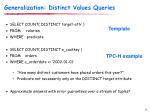Generalization: Distinct Values Queries
• SELECT COUNT( DISTINCT target-attr )
• FROM relation
• WHERE predicate
• SELECT COUNT( DISTINCT o_custkey )
• FROM orders
• WHERE o_orderdate >= ‘2002-01-01’
• “How many distinct customers have placed orders this year?”
• Predicate not necessarily only on the DISTINCT target attribute
• Approximate answers with error guarantees over a stream of tuples?

Template

TPC-H exampleDistinct Sampling [Gib01]

Key Ideas

• Use FM-like technique to collect a specially-tailored sample over the distinct values in the stream
• Use hash function mapping to sample values from the data domain!!
• Uniform random sample of the distinct values
• Very different from traditional random sample: each distinct value is chosen uniformly regardless of its frequency
• DISTINCT query answers: simply scale up sample answer by sampling rate
• To handle additional predicates
• Reservoir sampling of tuples for each distinct value in the sample
• Use reservoir sample to evaluate predicateslsb(h(17))

insert(17)

count0

count5

count6

count4

count2

count1

count7

TotCount

count3

+1

+1

+1

Processing Set Expressions over Update Streams [GGR03]
• Estimate cardinality of general set expressions over streams of updates
• E.g., number of distinct (source,dest) pairs seen at both R1 and R2 but not R3? | (R1 R2) – R3 |
• 2-Level Hash-Sketch (2LHS) stream synopsis: Generalizes FM sketch
• First level: buckets with exponentially-decreasing probabilities (using lsb(h(x)), as in FM)
• Second level: Count-signature array (logN+1 counters)
• One “total count” for elements in first-level bucket
• logN “bit-location counts” for 1-bits of incoming elements

-1 for deletes!!

17 = 0 0 0 1 0 0 0 1Extensions
• Key property of FM-based sketch structures: Duplicate-insensitive!!
• Multiple insertions of the same value don’t affect the sketch or the final estimate
• Makes them ideal for use in broadcast-based environments
• E.g., wireless sensor networks (broadcast to many neighbors is critical for robust data transfer)
• Considine et al. ICDE’04; Manjhi et al. SIGMOD’05
• Main deficiency of traditional random sampling: Does not work in a Turnstile Model (inserts+deletes)
• “Adversarial” deletion stream can deplete the sample
• Exercise: Can you make use of the ideas discussed today to build a “delete-proof” method of maintaining a random sample over a stream??New stuff for today…
• A different sketch structure for multi-sets: The CountMin (CM) sketch
• The Sliding Window model and Exponential Histograms (EHs)
• Peek into distributed streamingThe CountMin (CM) Sketch
• Simple sketch idea, can be used for point queries, range queries, quantiles, join size estimation
• Model input at each node as a vector xi of dimension N, where N is large
• Creates a small summary as an array of w ╳ d in size
• Use d hash functions to map vector entries to [1..w]

W

d+xi[j]

h1(j)

+xi[j]

+xi[j]

hd(j)

+xi[j]

CM Sketch Structure
• Each entry in vector A is mapped to one bucket per row
• Merge two sketches by entry-wise summation
• Estimate A[j] by taking mink sketch[k,hk(j)]

j,xi[j]

d

w

[Cormode, Muthukrishnan ’05]CM Sketch Summary
• CM sketch guarantees approximation error on point queries less than e||A||1 in size O(1/e log 1/d)
• Probability of more error is less than 1-d
• Similar guarantees for range queries, quantiles, join size
• Hints
• Counts are biased! Can you limit the expected amount of extra “mass” at each bucket? (Use Markov)
• Use Chernoff to boost the confidence for the min{} estimate
• Food for thought: How do the CM sketch guarantees compare to AMS??Sliding Window Streaming Model
• Model
• At every time t, a data record arrives
• The record “expires” at time t+N (N is the window length)
• When is it useful?
• Make decisions based on “recently observed” data
• Stock data
• Sensor networksTime in Data Stream Models

Tuples arrive X1, X2, X3, …, Xt, …

• Function f(X,t,NOW)
• Input at time t: f(X1,1,t), f(X2,2,t). f(X3,3,t), …, f(Xt,t,t)
• Input at time t+1: f(X1,1,t+1), f(X2,2,t+). f(X3,3,t+1), …, f(Xt+1,t+1,t+1)
• Full history:f == identity
• Partial history: Decay
• Exponential decay: f(X,t, NOW) = 2-(NOW-t)*X
• Input at time t: 2-(t-1)*X1, 2-(t-2)*X2,, …, ½ * Xt-1,Xt
• Input at time t+1: 2-t*X1, 2-(t-1)*X2,, …, 1/4 * Xt-1, ½ *Xt, Xt+1
• Sliding window (special type of decay):
• f(X,t,NOW) = X if NOW-t < N
• f(X,t,NOW) = 0, otherwise
• Input at time t: X1, X2, X3, …, Xt
• Input at time t+1: X2, X3, …, Xt, Xt+1,Simple Example: Maintain Max
• Problem: Maintain the maximum value over the last N numbers.
• Consider all non-decreasing arrangements of N numbers (Domain size R):
• There are ((N+R) choose N) distinct arrangements
• Lower bound on memory required:log(N+R choose N) >= N*log(R/N)
• So if R=poly(N), then lower bound says that we have to store the last N elements (Ω(N log N) memory)Statistics Over Sliding Windows
• Bitstream: Count the number of ones [DGIM02]
• Exact solution: Θ(N) bits
• Algorithm BasicCounting:
• 1 + ε approximation (relative error!)
• Space: O(1/ε (log2N)) bits
• Time: O(log N) worst case, O(1) amortized per record
• Lower Bound:
• Space: Ω(1/ε (log2N)) bitsApproach: Temporal Histograms

Example: … 01101010011111110110 0101 …

Equi-width histogram:

… 0110 1010 0111 1111 0110 0101 …

• Issues:
• Error is in the last (leftmost) bucket.
• Bucket counts (left to right): Cm,Cm-1, …,C2,C1
• Absolute error <= Cm/2.
• Answer >= Cm-1+…+C2+C1+1.
• Relative error <= Cm/2(Cm-1+…+C2+C1+1).
• Maintain: Cm/2(Cm-1+…+C2+C1+1) <= ε (=1/k).Naïve: Equi-Width Histograms
• Goal: Maintain Cm/2 <= ε (Cm-1+…+C2+C1+1)

Problem case:

… 0110 1010 0111 1111 0110 1111 0000 0000 0000 0000 …

• Note:
• Every Bucket will be the last bucket sometime!
• New records may be all zeros For every bucket i, require Ci/2 <= ε (Ci-1+…+C2+C1+1)Exponential Histograms
• Data structure invariant:
• Bucket sizes are non-decreasing powers of 2
• For every bucket size other than that of the last bucket, there are at least k/2 and at most k/2+1 buckets of that size
• Example: k=4: (8,4,4,4,2,2,2,1,1..)
• Invariant implies:
• Assume Ci=2j, then
• Ci-1+…+C2+C1+1 >= k/2*(Σ(1+2+4+..+2j-1)) >= k*2j /2 >= k/2*Ci
• Setting k = 1/e implies the required error guarantee!Space Complexity
• Number of buckets m:
• m <= [# of buckets of size j]*[# of different bucket sizes] <= (k/2 +1) * ((log(2N/k)+1) = O(k* log(N))
• Each bucket requires O(log N) bits.
• Total memory:O(k log2 N) = O(1/ε * log2 N) bits
• Invariant (with k = 1/e) maintains error guarantee!EH Maintenance Algorithm

Data structures:

• For each bucket: timestamp of most recent 1, size = #1’s in bucket
• LAST: size of the last bucket
• TOTAL: Total size of the buckets

New element arrives at time t

• If last bucket expired, update LAST and TOTAL
• If (element == 1) Create new bucket with size 1; update TOTAL
• Merge buckets if there are more than k/2+2 buckets of the same size
• Update LAST if changed

Anytime estimate: TOTAL – (LAST/2)Example Run
• If last bucket expired, update LAST and TOTAL
• If (element == 1) Create new bucket with size 1; update TOTAL
• Merge two oldest buckets if there are more than k/2+2 buckets of the same size
• Update LAST if changed

Example (k=2):

32,16,8,8,4,4,2,1,1

32,16,8,8,4,4,2,2,1

32,16,8,8,4,4,2,2,1,1

32,16,16,8,4,2,1Lower Bound
• Argument: Count number of different arrangements that the algorithm needs to distinguish
• log(N/B) blocks of sizes B,2B,4B,…,2iB from right to left.
• Block i is subdivided into B blocks of size 2i each.
• For each block (independently) choose k/4 sub-blocks and fill them with 1.
• Within each block: (B choose k/4) ways to place the 1s
• (B choose k/4)log(N/B) distinct arrangementsLower Bound (continued)
• Example:
• Show: An algorithm has to distinguish between any such two arrangementsLower Bound (continued)

Assume we do not distinguish two arrangements:

• Differ at block d, sub-block b

Consider time when b expires

• We have c full sub-blocks in A1, and c+1 full sub-blocks in A2 [note: c+1<=k/4]
• A1: c2d+sum1 to d-1 k/4*(1+2+4+..+2d-1) = c2d+k/2*(2d-1)
• A2: (c+1)2d+k/4*(2d-1)
• Absolute error: 2d-1
• Relative error for A2:2d-1/[(c+1)2d+k/4*(2d-1)] >= 1/k = ε

bLower Bound (continued)

A2

Calculation:

• A1: c2d+sum1 to d-1 k/4*(1+2+4+..+2d-1) = c2d+k/2*(2d-1)
• A2: (c+1)2d+k/4*(2d-1)
• Absolute error: 2d-1
• Relative error:2d-1/[(c+1)2d+k/4*(2d-1)] >=2d-1/[2*k/4* 2d] = 1/k = ε

A1The Power of EHs
• Counter for N items = O(logN) space
• EH = e-approximate counter over sliding window of N items that requires O(1/ε * log2 N) space
• O(1/e logN) penalty for (approx) sliding-window counting
• Can plugin EH-counters to counter-based streaming methods  work in sliding-window model!!
• Examples: histograms, CM-sketches, …
• Complication: counting is now e-approximate
• Account for that in analysisData-StreamAlgorithmicsModel
• Approximate answers– e.g. trend analysis, anomaly detection
• Requirements for stream synopses
• Single Pass: Each record is examined at most once
• Small Space: Log or polylog in data stream size
• Small-time: Low per-record processing time (maintain synopses)
• Also: delete-proof, composable, …

(Terabytes)

Stream Synopses

(in memory)

(Kilobytes)

Continuous Data Streams

R1

with Error Guarantees

“Within 2% of exact

probability”

Stream Processor

Rk

Query QQuery

Query site

Q(S1∪S2∪…)

S1

S3

S6

1

1

1

1

1

1

1

1

1

1

0

0

0

0

1

0

1

S5

S2

S4

0

1

0

0

1

Distributed Streams Model
• Large-scale querying/monitoring: Inherently distributed!
• Streams physically distributed across remote sitesE.g., stream of UDP packets through subset of edge routers
• Challenge is “holistic” querying/monitoring
• Queries over the union of distributed streams Q(S1 ∪S2 ∪ …)
• Streaming data is spread throughout the network

Network Operations

Center (NOC)Query

Query site

Q(S1∪S2∪…)

S1

S3

S6

1

1

1

1

1

1

1

1

1

1

0

0

0

0

1

0

1

S5

S2

S4

0

1

0

0

1

Distributed Streams Model
• Need timely, accurate, and efficient query answers
• Additional complexity over centralized data streaming!
• Need space/time- and communication-efficient solutions
• Minimize network overhead
• Maximize network lifetime (e.g., sensor battery life)
• Cannot afford to “centralize” all streaming data

Network Operations

Center (NOC)Conclusions
• Querying and finding patterns in massive streams is a real problem with many real-world applications
• Fundamentally rethink data-management issues under stringent constraints
• Single-pass algorithms with limited memory resources
• A lot of progress in the last few years
• Algorithms, system models & architectures
• GigaScope (AT&T), Aurora (Brandeis/Brown/MIT), Niagara (Wisconsin), STREAM (Stanford), Telegraph (Berkeley)
• Commercial acceptance still lagging, but will most probably grow in coming years
• Specialized systems (e.g., fraud detection, network monitoring), but still far from “DSMSs”